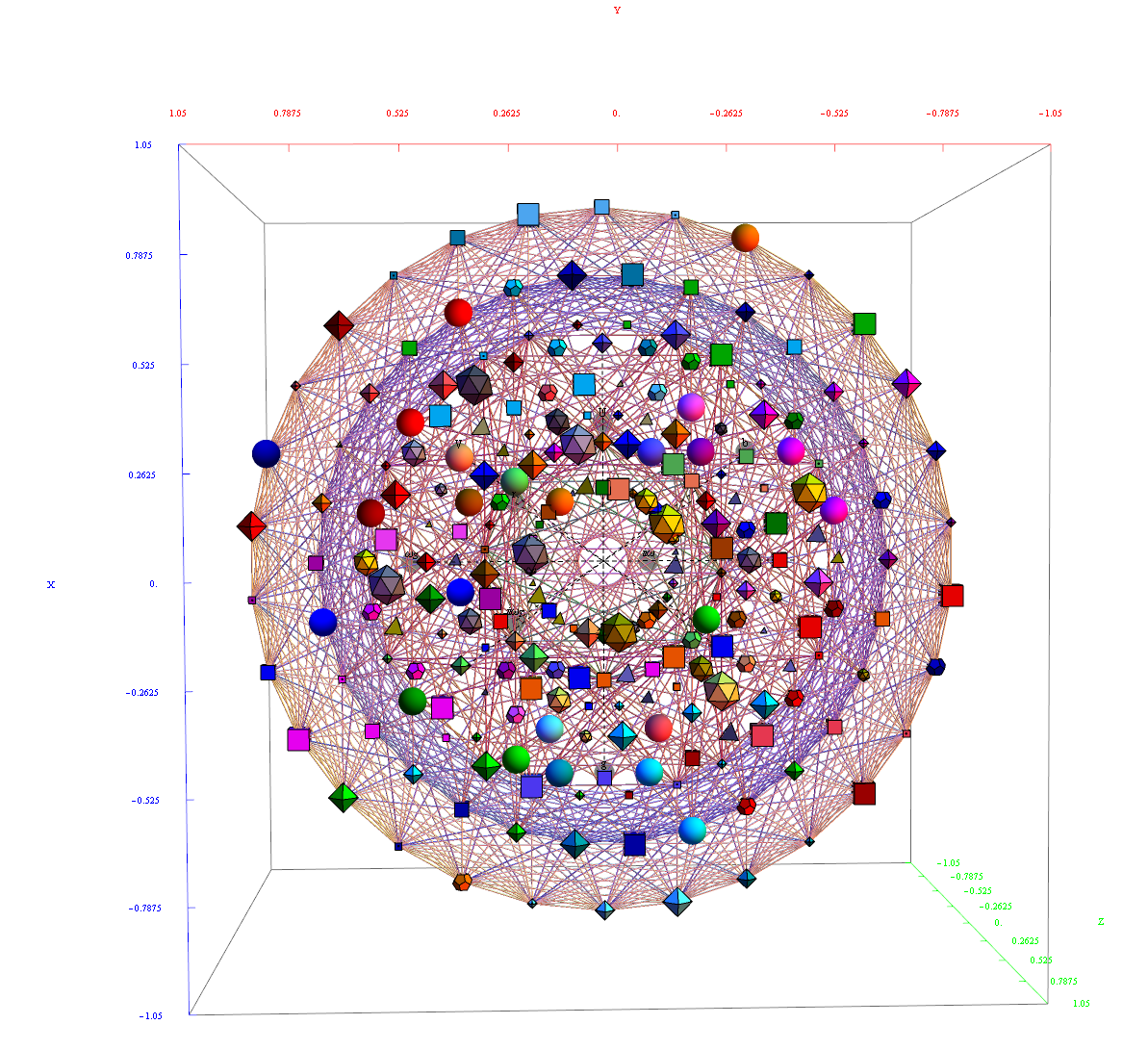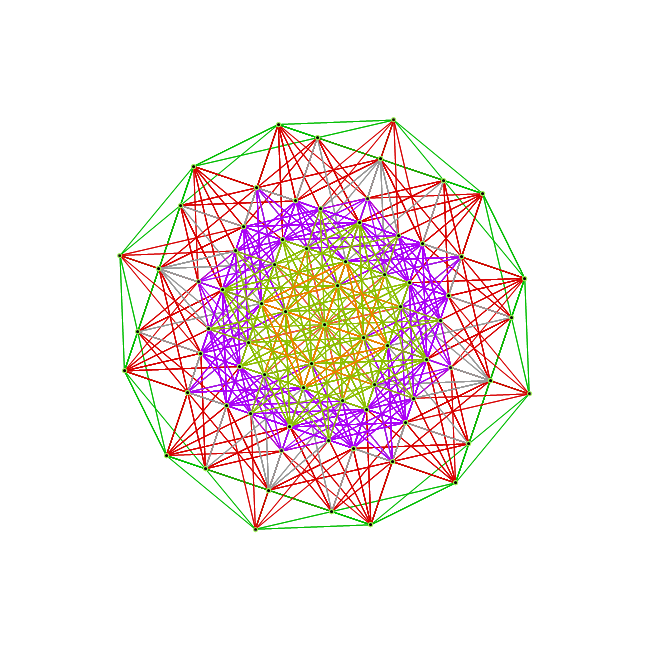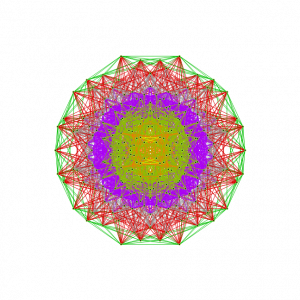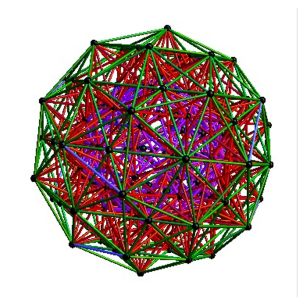# My latest paper on E8, the H4 folding matrix, and integration with octonions and GraviGUT physics models.

The full paper with appendix can be found on this site, or w/o appendix on viXra. (13 pages, 14 figures, 20Mb). The 22 page appendix contains the E8 algebra roots, Hasse diagram, and a complete integrated E8-particle-octonion list.Abstract:
This paper will present various techniques for visualizing a split real even E8 representation in 2 and 3 dimensions using an E8 to H4 folding matrix. This matrix is shown to be useful in providing direct relationships between E8 and the lower dimensional Dynkin and Coxeter-Dynkin geometries contained within it, geometries that are visualized in the form of real and virtual 3 dimensional objects. A direct linkage between E8, the folding matrix, fundamental physics particles in an extended Standard Model GraviGUT, quaternions, and octonions is introduced, and its importance is investigated and described.

If you would like to cite this, you can use this BibTex format if you like (remove the LaTex href tag structure if you don’t use the hyperref package):

@article{Moxness2014:006,
title={{The 3D Visualization of E8 using an H4 Folding Matrix}},
author={Moxness, J. G.},
journal={href{http://vixra.org/abs/1411.0130}{www.vixra.org/abs/1411.0130}},
year={2014},
month = nov,
}

# The 3rd (Z) basis vector for Bathsheba & Wizzy’s 600 CellWhile the 3D model I used to create the 2D Van Oss projection isomorphic to E8 Petrie (and a beautiful pentagonal view), it was not the same as what was being used by Richter in his 3D “pre-Van-Oss” construction. Given my H (or x) and V (or y), the 3rd basis vector for this projections is most likely:
Z={0, -0.0801064, 0, 0.236818, 0, 0.0801064, 0, -0.236818}
which reproduces the Richter, Bathsheba and Wizzy’s 3D models. Interestingly, it produces one face (shown above) that is the same as all the orthonormal faces of 2 concentric 600 Cells (at the Golden Ratio). The 3rd unique face is:I replaced the 3D spin movie of this on my main page with this new projection.

# Dual 600 Cell (H4+H4/Phi) Petrie Projection to Square,Penta & Hexagon

I have published my ToE.pdf to vixra.org http://vixra.org/abs/1006.0063 .  While looking around vixra, I found a paper by Tony Smith on E8 http://vixra.org/pdf/0907.0006v1.pdf.  It has much of the work on his website including references to Lisi and Bathsheba on their visualizations of E8.  Some of the references are based on the 600 Cell set H4 & H4/Phi (shown by Richter to be isomorphic to E8). I can show the E8 Dynkin diagram can indeed be folded to H4.

The H4 and its 120 vertices make up the 4D 600 Cell (which is made up of 96 vertices of the snub-24 Cell and the 24 vertices of the 24 Cell=[16 vertex Tesseract=8-Cell and the 8 vertices of the 4-Orthoplex=16 Cell]). It can be generated from the 240 split real even E8 vertices using a 4×8 rotation matrix:
x = (1, φ, 0, -1, φ, 0, 0, 0)
y = (φ, 0, 1, φ, 0, -1, 0, 0)
z = (0, 1, φ, 0, -1, φ, 0, 0)
w = (0, 0, 0, 0, 0, 0, φ^2, 1/φ)

where φ=Golden Ratio=(1+Sqrt(5))/2

I find in folding from 8D to 4D, that the 6720 edge counts split into two sets of 3360 from E8’s 6720 length Sqrt(2).

I recreated here a E8 Petrie projection which is isomorphic to 2D split real even E8 Petrie projection (except it only produces half of the 6720 “shortest edge” counts, of different length). All of these use a single set of 3 projection basis vectors to produce a single 3D projected object with 3 unique faces. On one face, it has the famous E8 Petrie projection:

On another face it has a pentagonal projection:

On the third unique face is the hexagonal projection:

I created a movie (similar to those of Lisi) of the H4+H4/Phi 600 Cells that rotates the 2D H-V E8 face projection to the H-Z Pentagonal face (shown in the middle frames of the movie). This is an interesting rotation because this rotates through a square projection at around Pi/8.

A lower quality YouTube is here: 600 Cells E8 to Pentagonal.  Download and play the higher quality .avi: cell600E8pent

I also created a 3D perspective spin of that projection (two sets of mutually orthogonal cubic faces). On YouTube:600 Cells 3D Spin.  Download the higher quality .avi: cell6003Dspin2

Similar to the work of other 3D artists like Bathsheba and Wizzy in Second Life (SL), this object can be created in Real Life (RL) as 3D laser etched crystal or rezzed as a 3000+ prim object in SL.

The 3 (H,V,Z) basis projection vectors are: